Select Page

# Seminar in Mathematical Physics

In 2021, this seminar is a part of the EIMI thematic program “Spectral Theory and Mathematical Physics“. The meetings are held weekly on Wednesdays at 18:30 Moscow Time (15:30 UTC) unless otherwise noted. To subscribe to our mailing list and receive zoom meeting information, please sign up online.

The seminar is organized by the Department of Mathematics and Mathematical Physics at SPbU within the program “Spectral Theory and Mathematical Physics“. If you have any questions, please contact Tatiana Suslina

## Operator-norm asymptotics for thin elastic rods with rapidly oscillating periodic properties

We provide norm-resolvent estimates in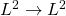andoperator norms, for the class of problems in linear elasticity describing heterogeneous rods with rapidly oscillating coefficients in the regime of moderate contrast.

The estimates are provided with respect to the period of material oscillations in the setting of simultaneous homogenization and dimension reduction, while assuming that the period and the rod thickness are of the same order. These estimates are expected to provide also sharp estimates for the corresponding evolution problems.

The analysis is performed by the means of spectral analysis and Gelfand transform.

This is joint work with K.Cherednichenko and I.Velčić.

## Differential operators with constant coefficients and formulas for the sums of some converging numerical series

The lecture will present a method that allows, using the spectral theory of ordinary self-adjoint differential operators with constant coefficients, to find integral representations for the sums of some converging series, and calculate some of them in terms of elementary functions. This method makes it possible, for example, to write in terms of elementary functions general formulas for the sums of numerical series, the kth term of which is one over the value at the point k of a polynomial with real coefficients of degree greater than one, which has no zeros on the integer lattice. In addition, our method leads to various generating functions for the values ​​of the Riemann zeta function and related functions at natural points, which allow us to uniformly prove the well-known formulas for the values ​​of the zeta function at odd numbers and obtain some new ones. In this way, in particular, it is possible to obtain new representations for the constants Catalan, Apéry, and ln 2.

## On the semiclassical approximation for nonlocal operators

The talk deals with two situations: 1) Difference equations on a multidimensional lattice with a small step. 2) Differential equations with a small parameter multiplying the derivatives on a compact manifold with
coefficients that include the action of a discrete group of translations. In both cases, a construction of semiclassical asymptotic solutions based on the Maslov canonical operator is presented.

This work was supported by the RFBR grant No. 21-51-12006.

## Lieb–Thirring estimates for Schrödinger operators with singular measures as potential

L–T estimates for the Schrödinger operator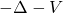in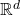concern the sum of powers of moduli of negative eigenvalues,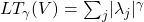. The standard L–T estimate has the form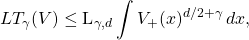which holds for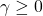,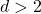;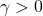,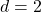;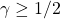,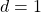. These estimates play important role in the spectral theory of quantum, especially many-particle, systems and were being under active development during latest 50 years. In the talk we discuss some recent results on the L–T inequalities for Schrödinger-like operators with potential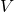replaced by a measure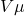, where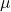is a measure singular with respect to the Lebesgue measure andis a-measurable function. Typical measuresin this setting are the surface measure on a Lipschitz surface of dimension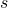inor the Hausdorff measure on a fractal set. The general estimate for the operator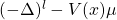has the form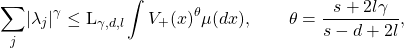whereis the dimensional characteristic of the measure.

## Возмущения оператора Мёлера и его применение к функционально-разностным уравнениям с мероморфным потенциалом

В докладе рассматривается возмущенный оператора Мёлера, который естественным образом возникает при изучении функционально-разностных уравнений второго порядка с мероморфным потенциалом, зависящими от характеристического параметра (константы связи). В частности, такого рода функционально-разностные уравнения встречаются при построении асимптотики (по расстоянию) собственных функций лапласианов с сингулярным потенциалом, имеющим носитель на клиновидных или конусообразных поверхностях.

Невозмущенный самосопряженный оператор Мёлера – интегральный оператор в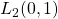с ядром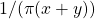, который изучается с помощью модифицированного преобразования Мёлера-Фока. Рассматриваются его резольвента и спектральная мера. В конце доклада, при наличии времени, кратко обсудим способы получения достаточных условий существования дискретного спектра для класса компактных возмущенийэтого оператора, т.е. для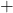, а также возможности применения принципа Бирмана-Швингера для получения оценки числа собственных значений, расположенных справа от существенного спектра. В последнем случае, подход аналогичен, предложенному Д.Р.Яфаевым (2014 г.) для возмущений оператора Карлемана операторами Ханкеля.

## Bethe–Sommerfeld property of periodic and almost-periodic Schrödinger operators: results old and new

I will give a survey of situations when we can establish a Bethe–Sommerfeld property (that all large energies belong to the spectrum) for multidimensional Schrödinger operators with periodic and almost-periodic potentials. While in the periodic setting the results are more than ten years old, there has been substantial recent activity in the almost-periodic case. In particular, I will describe my recent joint result with Yu.Karpeshina and R.Shterenberg.

## New effective integral representations for the canonical operator, special functions and applications to wave beams and the theory of linear water waves

The canonical Maslov operator is one of the powerful tools for constructing semiclassical asymptotics. Classical objects arising in the canonical operator are Lagrangian manifolds in phase space, and the features of their projection onto the configuration (physical) space are caustics, focal points, and other Lagrangian singularities. In their neighborhood, the asymptotics determined by the canonical operator is represented as an integral over the impulse variables (or part of them). The representation recently proposed by S.Y.Dobrokhotov, V.E.Nazaikinsky and A.I.Shafarevich relies on integration in the corresponding domains (charts) with respect to some coordinates on a Lagrangian manifold directly. This representation significantly simplifies the construction of the canonical operator and allows us to expand its scope to frequently encountered problems with non-smooth Lagrangian manifolds. In addition, these representations for a number of problems allow us to write uniform asymptotic solutions in the form of special functions of a complex argument in a wide neighborhood of caustics. As applications, we consider examples from the theory of wave beams and the linear theory of waves on water.

This talk is based on joint work with V.E.Nazaikinskii, A.I.Shafarevich, A.Yu.Anikin, D.S.Minenkov, A.A.Tolchennikov and A.V.Tsvetkova.

## Feynmann–Maslov calculus of functions of noncommuting operators and applications to adiabatic and semiclassical problems. Part 2

At the elementary level, we discuss the basic objects and formulas in the Feynman–Maslov operator theory about functions of non-commuting operators. The main objects here are differential and pseudo-differential operators with a small parameter (h-pseudo-differential operators). As a simple effective application, we consider the use of this theory in adiabatic problems (in particular, in dimension reduction problems). These include vector problems (for example, problems about wave functions in graphene), problems about wave propagation in waveguides (for example, waves in nanotubes), problems for equations with rapidly changing coefficients (averaging methods), etc. As a result of the application of the considered approach (formulated in the form of an algorithm), the initial problems are reduced to simpler problems described by “effective” Hamiltonians or modes, and containing, in particular, dispersion effects. Then one can use the semi-classical approximation to construct asymptotic solutions of the reduced equations.

The first part of the lectures is devoted to elementary definitions and important effective formulas of operator calculus and applications to vector problems (the simplest problems with an “operator-valued symbol”). The second part is devoted to the more complicated adiabatic problems mentioned above.

## Feynmann–Maslov calculus of functions of noncommuting operators and applications to adiabatic and semiclassical problems. Part 1

At the elementary level, we discuss the basic objects and formulas in the Feynman–Maslov operator theory about functions of non-commuting operators. The main objects here are differential and pseudo-differential operators with a small parameter (h-pseudo-differential operators). As a simple effective application, we consider the use of this theory in adiabatic problems (in particular, in dimension reduction problems). These include vector problems (for example, problems about wave functions in graphene), problems about wave propagation in waveguides (for example, waves in nanotubes), problems for equations with rapidly changing coefficients (averaging methods), etc. As a result of the application of the considered approach (formulated in the form of an algorithm), the initial problems are reduced to simpler problems described by “effective” Hamiltonians or modes, and containing, in particular, dispersion effects. Then one can use the semi-classical approximation to construct asymptotic solutions of the reduced equations.

The first part of the lectures is devoted to elementary definitions and important effective formulas of operator calculus and applications to vector problems (the simplest problems with an “operator-valued symbol”). The second part is devoted to the more complicated adiabatic problems mentioned above.

## Subordination theory for Jacobi matrices: Is there any analog for block Jacobi operators?

The notion of subordinacy was first introduced by Gilbert and Pearson (1987) for self-adjoint continuous Shrödinger operators on half-line. Soon it was formulated also for the discrete case – for self-adjoint Jacobi operators (Pearson and Khan, 1992).

Subordination theory joins the spectral problems for the operator (related to its absolutely continuous and the singular parts) with some specific “asymptotic” properties of the solutions from the 2-dimensional spaces of the generalized eigensolutions for the spectral parameters λ ∈ ℝ.

I will talk about some main hopes and questions concerning my joint studies with Grzegorz Świderski on formulating an analog of subordination theory for s.a. block Jacobi operators. I would like to recall first the main steps and ideas of the proof of Khan–Pearson theorem. Then I will show which steps and important relations of the scalar case have some “good” analogs in the block case.

## Semi-classical limit and least action principle revisited with (min,+) path integral and action-particle duality

We would like to present how the idempotent algebra (min,+) and its analysis, constituting a natural and well-suited framework to study the semi-classical limit of quantum mechanics.
Idempotent mathematics in general, and (min, +) one in particular, are well-known to handle in a linear way non-linear problems occurring for example in informatics, applied mathematics and nowadays in theoretical physics as well.

One will define the so-called (min, +) Path Integral, an analogous of Feynman’s Path Integral in quantum physics, but designed for classical mechanics. The Hamilton-Jacobi equations and their solutions within this mathematical framework, are introduced and yield to a new interpretation expressed in a duality between action field and particle.

The talk is based on joint work with Michel Gondran and Alexandre Gondran.

## On the spectrum of the Kronig-Penney model in a constant electric field

We are interested in the nature of the spectrum of the one-dimensional Schrödinger operator whose potential is a combination of the linear Stark potential and an equidistant arrangement of delta functions. When all the coupling constants of the delta functions are equal and the strength of the electric field satisfies a rationality condition, we show that the spectrum is purely absolutely continuous away from an explicit discrete set of points. On the other hand, when the coupling constants are iid random variables, we prove that there is a transition between purely singularly continuous spectrum and purely pure point spectrum at a transition point that we compute explicitly. These models have been discussed in the solid state physics literature and our results in the first model confirms predictions by Buslaev.

The talk is based on joint work with S. Larson.

## Homogenization of spin energies on Poisson random process

We consider nearest-neighbour ferromagnetic spin energies defined on a Poisson random process in the plane and show that their discrete-to-continuous scaling limit is an isotropic perimeter energy with a surface tension characterised by asymptotic characteristics of the Poisson random process.

## Invariant subspaces of elliptic systems

Consider an elliptic self-adjoint pseudodifferential operator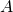acting on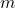-columns of half-densities on a closed manifold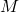, whose principal symbol is assumed to have simple eigenvalues. We show existence and uniqueness oforthonormal pseudodifferential projections commuting with the operatorand provide an algorithm for the computation of their full symbols, as well as explicit closed formulae for their subprincipal symbols. Pseudodifferential projections yield a decomposition of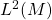into invariant subspaces under the action ofmodulo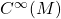. Furthermore, they allow us to decomposeintodistinct sign definite pseudodifferential operators.

We use our pseudodifferential projections to show that the spectrum ofdecomposes, up to an error with superpolynomial decay, intodistinct series, each associated with one of the eigenvalues of the principal symbol of. These spectral results are then applied to the study of propagation of singularities in hyperbolic systems.

This is joint work with Matteo Capoferri (Cardiff). The talk is based on two of our papers, arXiv:2103.14325 and arXiv:2103.14334.

## On negative eigenvalues of the spectral problem for water waves of highest amplitude

We consider a spectral problem connected with steady water waves of highest amplitude on the free surface of rotational flow. It is proved that the spectrum of this problem contains arbitrary large negative eigenvalues and they are simple. Moreover the asymptotics of such eigenvalues is obtained. This is a joint work with E.Lokharu, Linköping University.

(This is a joint session with V.I.Smirnov Seminar on Mathematical Physics.)

## Multi-mode quantum graphs – a new approach (and results) of old and new problems

We return to an old problem – the Schrӧdinger operator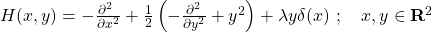– a paradigm model for multi-mode quantum graphs, and discuss it using a scattering approach. This will enable shedding some light on previous results, and study some other related operators, e.g., the period case – the analogous Kronig Penney model.

## Convergence of the form factor series in the Sinh-Gordon quantum field theory in 1+1 dimensions

Within the approach of the bootstrap program, the physically pertinent observables in a massive integrable quantum field theory in 1+1 dimensions are expressed by means of the so-called form factor series expansion. This corresponds to a series of multiple integrals in which the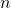th summand is given by a-fold integral. While being formally effective for various physical applications, so far, the question of convergence of such form factor series expansions was essentially left open. Still, convergence results are necessary so as to reach the mathematical well-definiteness of such construction and appear as necessary ingredients for the justification of numerous handlings that are carried out on such series and which play the role in the analysis of the physics at the roots of such models.

In this talks, I will first go through the physical origin as well as the various motivations for studying the convergence of form factor series expansions in massive quantum integrable field theories. Then, I will provide a description of the problem per se, in particular by discussing the Sinh-Gordon quantum field theory in 1+1 dimensions within the bootstrap program approach. Once this is settled, if time permits, I will discuss the the main features of the technique that allows one to prove this convergence. The proof amounts to obtaining a sufficiently sharp estimate on the leading large-behaviour of the-fold integral arising in this context. This appeared possible by refining some of the techniques that were fruitful in the analysis of the large-behaviour of integrals over the spectrum of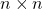random Hermitian matrices.

## Geodesic beams and Weyl remainders

In this talk we discuss quantitative improvements for Weyl remainders under dynamical assumptions on the geodesic flow. We consider a variety of Weyl type remainders including asymptotics for the eigenvalue counting function as well as for the on and off diagonal spectral projector. These improvements are obtained by combining the geodesic beam approach to understanding eigenfunction concentration together with an appropriate decomposition of the spectral projector into quasimodes for the Laplacian. One striking consequence of these estimates is a quantitatively improved Weyl remainder on all product manifolds. This is joint work with Y.Canzani.

## Virtual levels and virtual states of operators in Banach spaces

Virtual levels admit several equivalent characterizations:
(1) there are corresponding eigenstates from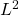or a space “slightly weaker” than;
(2) there is no limiting absorption principle in the vicinity of a virtual level (e.g. no weights such that the “sandwiched” resolvent remains uniformly bounded);
(3) an arbitrarily small perturbation can produce an eigenvalue.
We develop a general approach to virtual levels in Banach spaces and provide applications to Schroedinger operators with nonselfadjoint potentials and in any dimension.

This is a joint work with Nabile Boussaid based on the preprint

## On the absolute continuity of Toeplitz operators

It has been known since the 1960’s that the spectra of Toeplitz operators are purely absolutely continuous. The aim of the talk is to give a short survey of relevant results and to describe a new spectral classification for Toeplitz operators with piecewise continuous symbols. The classification result is obtained by analysing scattering properties of Toeplitz operators.

The talk is based on a joint work with D.Yafaev (Rennes).

## Szegő-type limit theorems for “multiplicative Toeplitz” operators and non-Følner approximations

We will discuss an analogue of the First Szegő Limit Theorem for multiplicative Toeplitz operators and highlight the role of the multliplicative Følner condition in this topic.

The talk is based on a joint work with Nikolai Nikolski.

## The Birman–Schwinger type operator with singular measure. Eigenvalues analysis, Connes integral and rectifiable sets

This is an extended version of the authors’ talk on 28.12.2020 at the V.I.Smirnov seminar, containing some new results. We consider the Birman–Schwinger type operator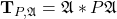, where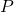is a signed measure in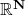and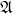is a pseudodifferential operator inof order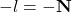(in the leading case,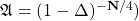. Under rather general conditions we find eigenvalue estimates for this operator, and for measures supported on a Lipschitz surface, find eigenvalue asymptotics. The interesting case is when measurecontains a singular component. A peculiar feature here is that the order of the eigenvalue estimates and asymptotics does not depend on the dimensional characteristics of the support of the measure, so, contributions of components of different dimensions just add up. Further on the results are carried over to more general measures supported on so-called rectifiable sets. We will discuss relation of our results to spectral theory of fractals, logarithmic potential and, finally, to noncommutative integration of singular measures.

## Whispering gallery waves near boundary inflection: a canonical high-frequency diffraction problem

A particular problem of interest is that of a whispering gallery high-frequency asymptotic mode propagating along a concave part of a boundary and then scattering at a boundary inflection point. Like Airy ODE and associated Airy function are fundamental for describing transition from oscillatory to exponentially decaying asymptotic behaviors, the boundary inflection problem leads to an arguably equally fundamental canonical boundary-value problem for a special PDE describing transition from a “modal” to a “scattered” high-frequency asymptotic behaviors. This is a Schrödinger equation on a half-line with a potential linear in both space and time. The latter problem was first formulated and analysed by M.M.Popov starting from 1970-s. The associated solutions have asymptotic behaviors with a discrete spectrum at one end and with a continuous spectrum at the other end, and of central interest is to find the map connecting the above two asymptotic regimes. The problem however lacks separation of variables, except in the asymptotical sense at both of the above ends.

Nevertheless, we perform a non-standard perturbation analysis at the continuous spectrum end, ultimately describing the desired map connecting the two asymptotic representations. We also show that the problem permits a re-formulation as a one-dimensional boundary integral equation, whose regularization allows its further asymptotic analysis.

## Real-valued semiclassical approximation for the asymptotics with complex-valued phases for Plancherel-Rotach asymptotics of Hermitian type orthogonal polynomials

The Hermitian type orthogonal polynomials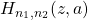are determined by the pair of recurrence relations for the polynomials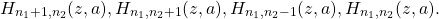We obtain a uniform asymptotics of diagonal polynomials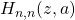in the form of an Airy function for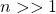, which is a far-reaching generalization of the Plancherel-Rotach asymptotic formulas for Hermitian polynomials. We discuss one of the possible approach which we call “real-valued semiclassics for asymptotics with complex-valued phases” (another approach based on the construction of decompositions of bases of homogeneous difference equations was recently developed by A.I.Aptekarev and D.N.Tulyakov). This approach can be applied to construct asymptotic formulas for various orthogonal polynomials. Introducing an artificial small parameter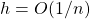and a continuous function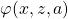such that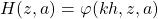, we reduce the described to a pseudo-differential equation for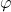, where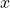is a variable and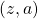are parameters. Seeking its solution in the WKB-form, one obtains the Hamilton-Jacobi equations with complex Hamiltonians connected with a third-order algebraic curve. This circumstance is the main difficulty of solving the problem and, as a rule, leads to the transition from the real variableto the complex one. In this problem, we propose a different approach based on a reduction of the original problem to three equations, two of which have asymptotics with a purely imaginary phase, and the symbol of the third one is pure real and has the form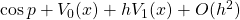. This ultimately allows us to represent the desired asymptotic uniformly through the Airy function of the complex but real-valued argument.

The talk is based on a joint work with A.I.Aptekarev (Keldysh Institute of Applied Mathematics RAS), D.N.Tulyakov (Keldysh Institute of Applied Mathematics RAS) and A.V.Tsvetkova (Ishlinsky Institute for Problems in Mechanics RAS).